Chapter 7 Class 10 Coordinate Geometry

Class 10
Important Questions for Exam - Class 10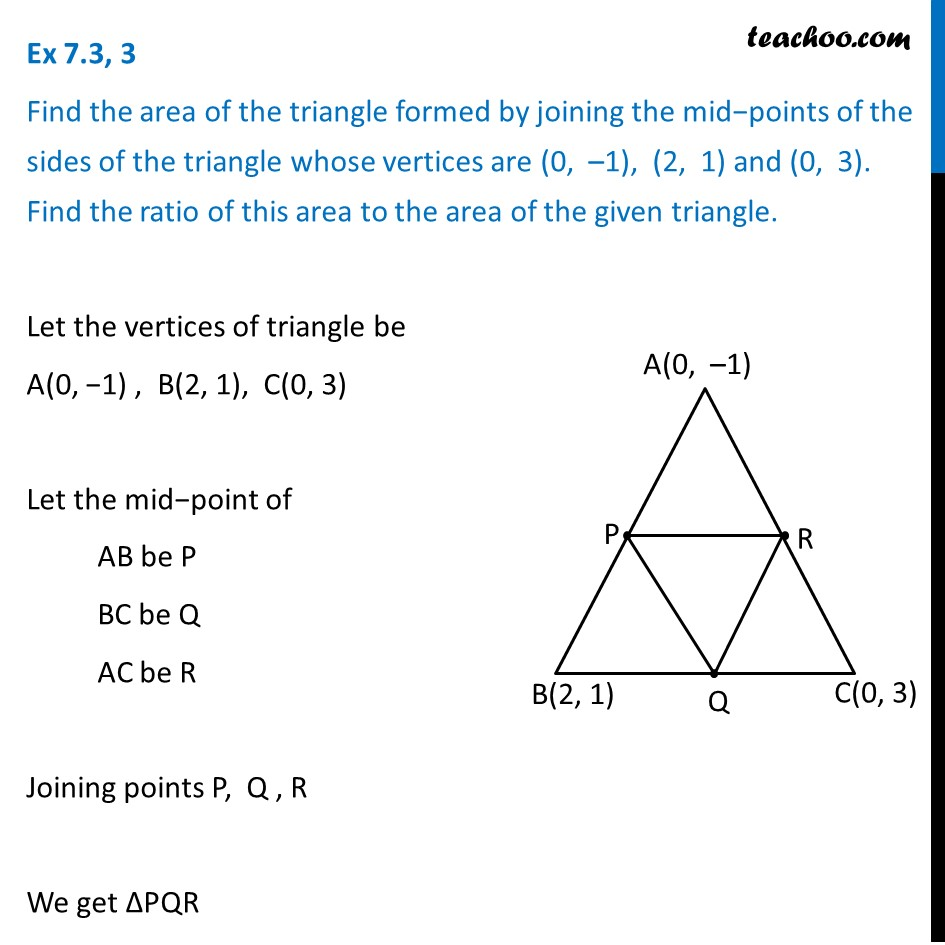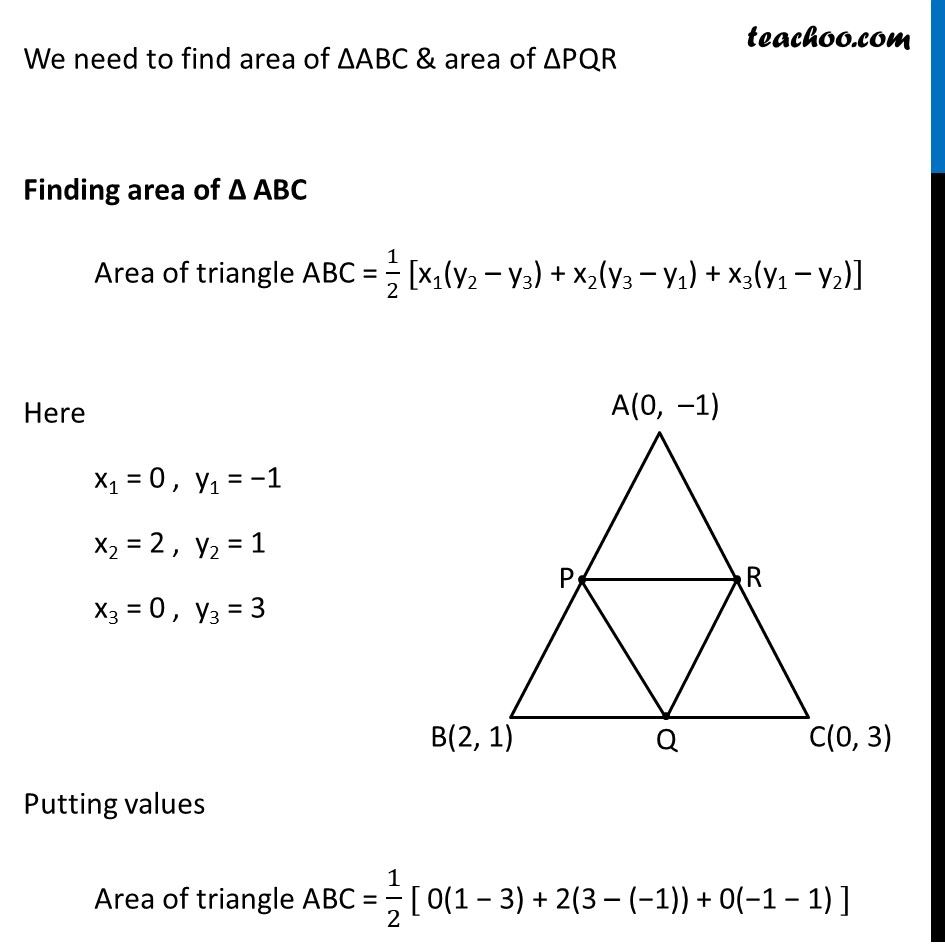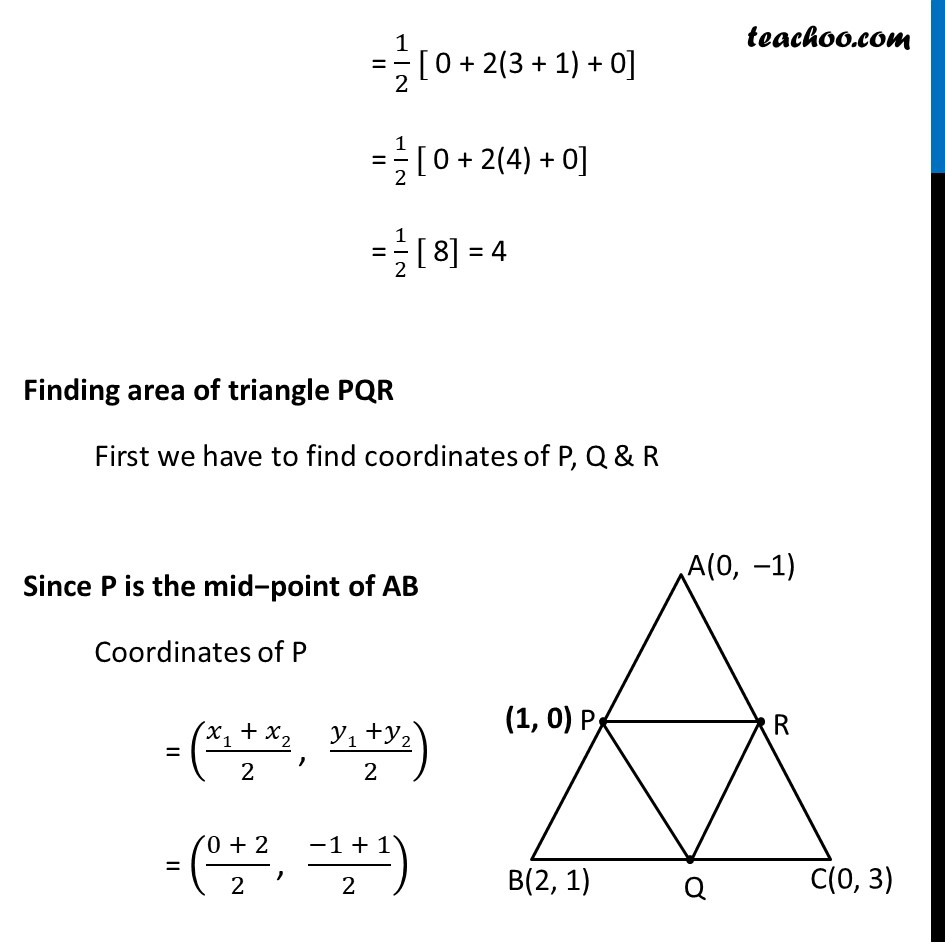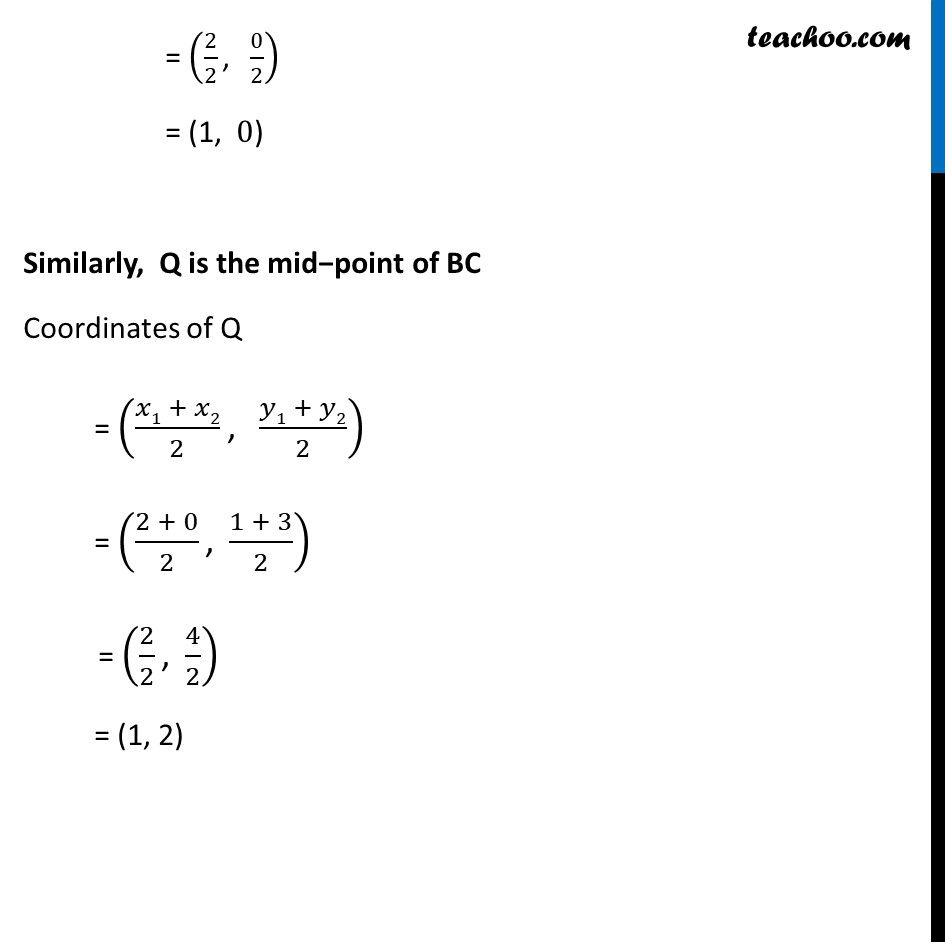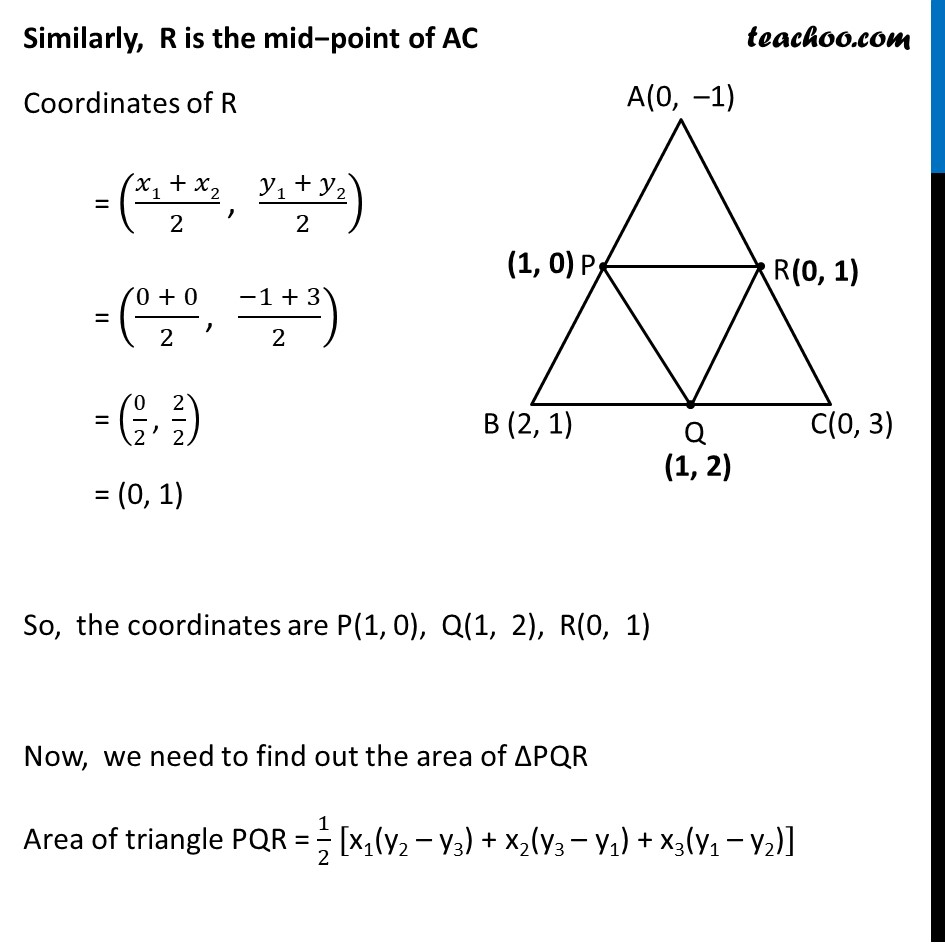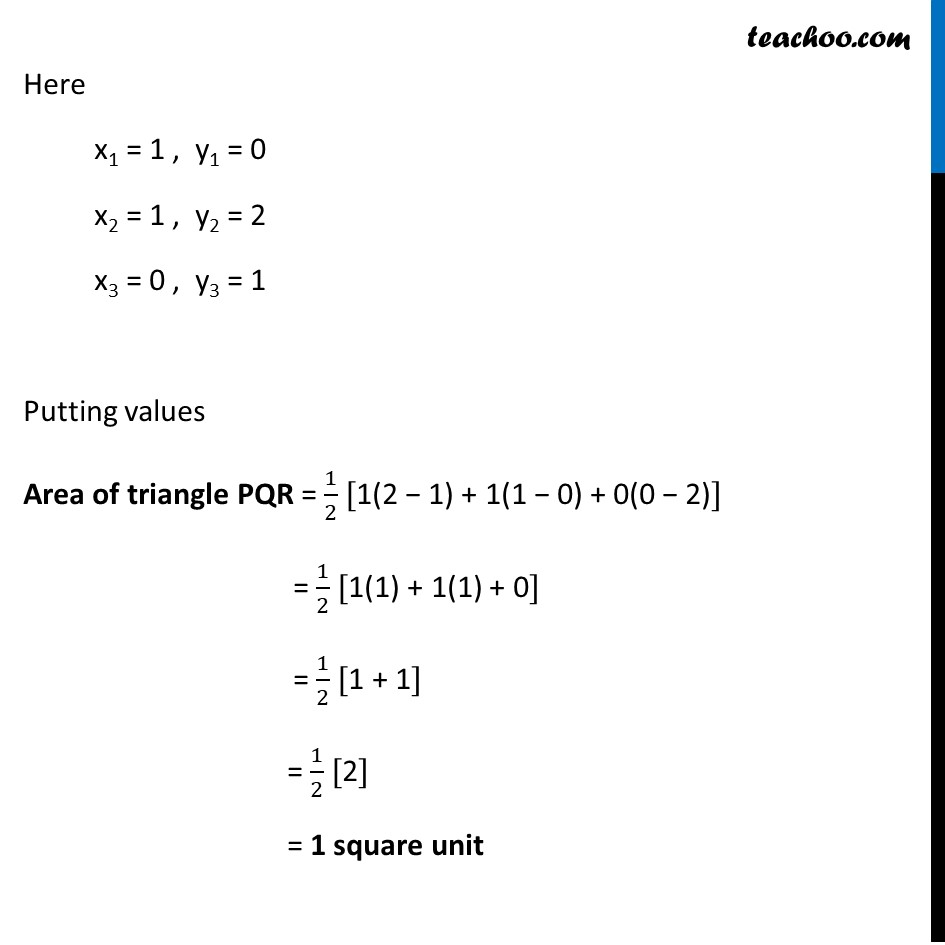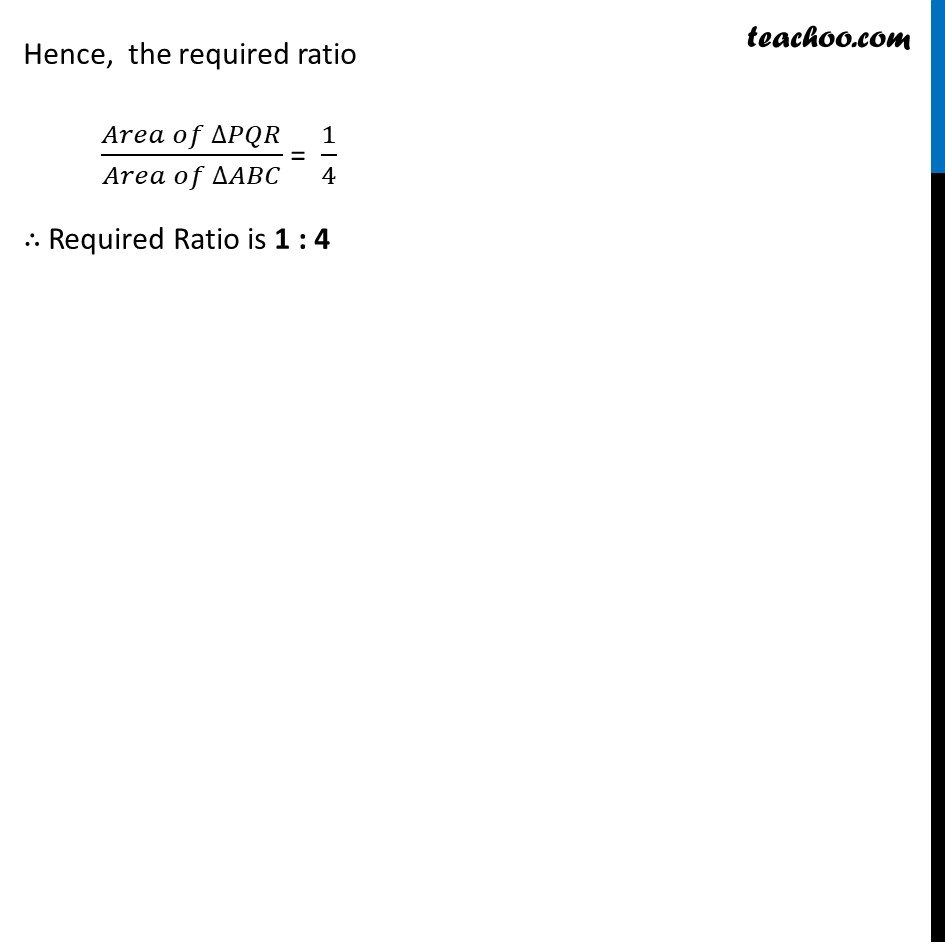Introducing your new favourite teacher - Teachoo Black, at only ₹83 per month

### Transcript

Ex 7.3, 3 Find the area of the triangle formed by joining the mid−points of the sides of the triangle whose vertices are (0, –1), (2, 1) and (0, 3). Find the ratio of this area to the area of the given triangle. Let the vertices of triangle be A(0, −1) , B(2, 1), C(0, 3) Let the mid−point of AB be P BC be Q AC be R Joining points P, Q , R We get ∆PQR We need to find area of ∆ABC & area of ∆PQR Finding area of ∆ ABC Area of triangle ABC = 1/2 [x1(y2 – y3) + x2(y3 – y1) + x3(y1 – y2)] Here x1 = 0 , y1 = −1 x2 = 2 , y2 = 1 x3 = 0 , y3 = 3 Putting values Area of triangle ABC = 1/2 [ 0(1 − 3) + 2(3 – (−1)) + 0(−1 − 1) ] = 1/2 [ 0 + 2(3 + 1) + 0] = 1/2 [ 0 + 2(4) + 0] = 1/2 [ 8] = 4 Finding area of triangle PQR First we have to find coordinates of P, Q & R Since P is the mid−point of AB Coordinates of P = ((𝑥1 + 𝑥2)/2 ", " (𝑦1 +𝑦2)/2) = ((0 + 2)/2 ", " (−1 + 1)/2) = (2/2 ", " 0/2) = (1, 0) Similarly, Q is the mid−point of BC Coordinates of Q = ((𝑥1 + 𝑥2)/2 ", " (𝑦1 + 𝑦2)/2) = ((2 + 0)/2 ", " (1 + 3)/2) = (2/2 ", " 4/2) = (1, 2) Similarly, R is the mid−point of AC Coordinates of R = ((𝑥1 + 𝑥2)/2 ", " (𝑦1 + 𝑦2)/2) = ((0 + 0)/2 ", " (−1 + 3)/2) = (0/2 ", " 2/2) = (0, 1) So, the coordinates are P(1, 0), Q(1, 2), R(0, 1) Now, we need to find out the area of ∆PQR Area of triangle PQR = 1/2 [x1(y2 – y3) + x2(y3 – y1) + x3(y1 – y2)] Here x1 = 1 , y1 = 0 x2 = 1 , y2 = 2 x3 = 0 , y3 = 1 Putting values Area of triangle PQR = 1/2 [1(2 − 1) + 1(1 − 0) + 0(0 − 2)] = 1/2 [1(1) + 1(1) + 0] = 1/2 [1 + 1] = 1/2  = 1 square unit Hence, the required ratio (𝐴𝑟𝑒𝑎 𝑜𝑓 ∆𝑃𝑄𝑅)/(𝐴𝑟𝑒𝑎 𝑜𝑓 ∆𝐴𝐵𝐶) = 1/4 ∴ Required Ratio is 1 : 4